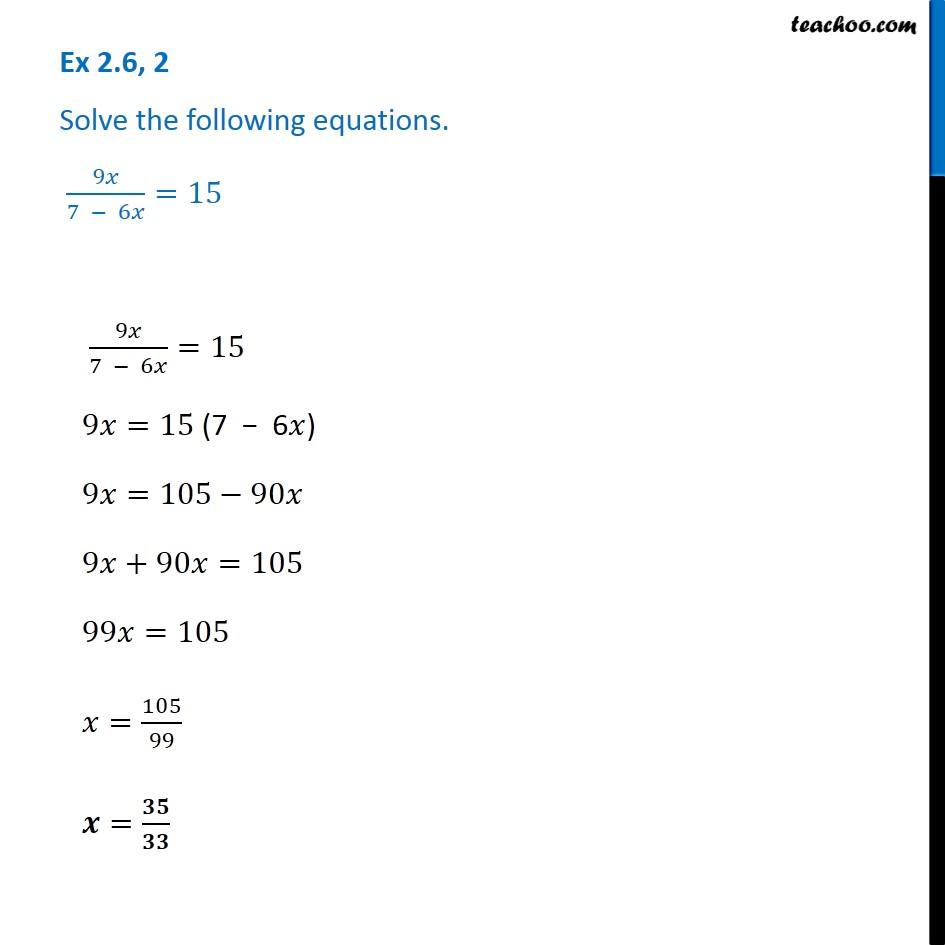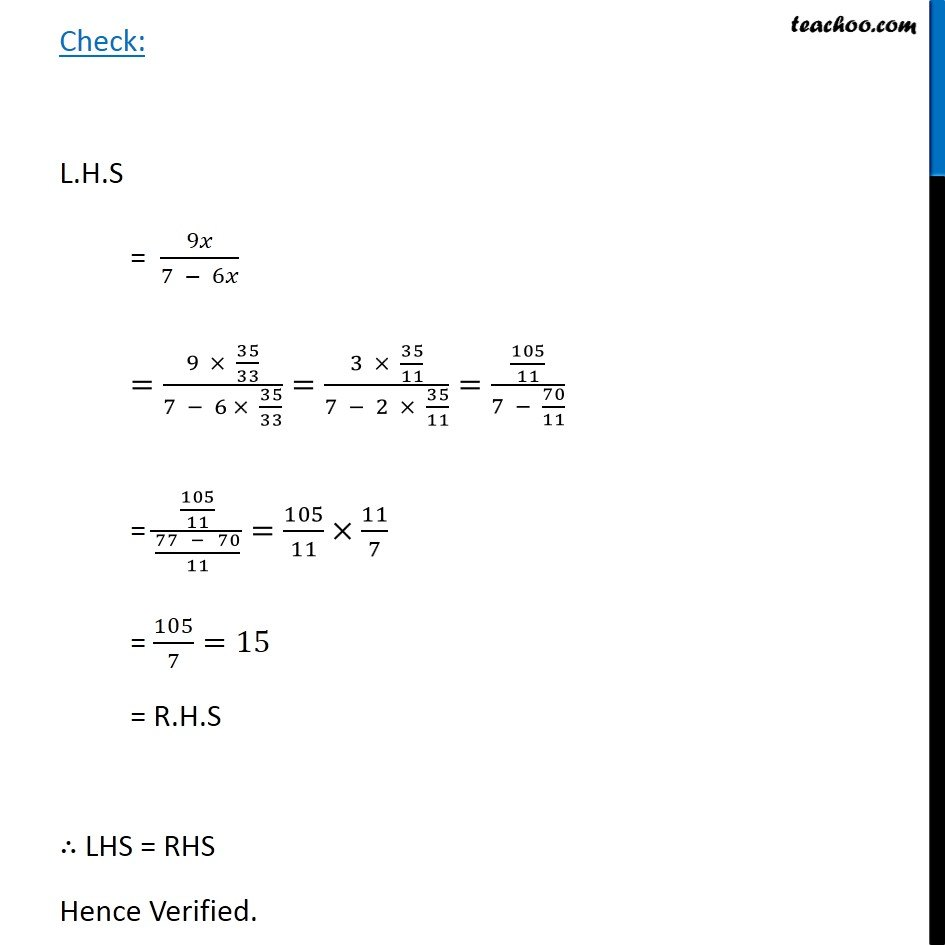Solving difficult equations - Word Problems

Chapter 2 Class 8 Linear Equations in One Variable
Serial order wiseLearn in your speed, with individual attention - Teachoo Maths 1-on-1 Class

### Transcript

Question 2 Solve the following equations. 9 /(7 6 )=15 9 /(7 6 )=15 9 =15 (7 6 ) 9 =105 90 9 +90 =105 99 =105 =105/99 = / Check: L.H.S = 9 /(7 6 ) =(9 35/33)/(7 6 35/33)=(3 35/11)/(7 2 35/11)=(105/11)/(7 70/11) = (105/11)/((77 70)/11)=105/11 11/7 = 105/7=15 = R.H.S LHS = RHS Hence Verified.